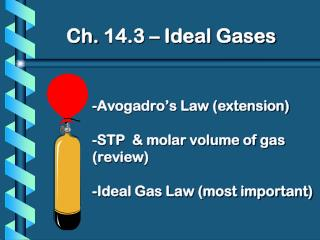DownloadDownload Presentation-Avogadro’s Law (extension) -STP & molar volume of gas (review) -Ideal Gas Law (most important)

# -Avogadro’s Law (extension) -STP & molar volume of gas (review) -Ideal Gas Law (most important)

Télécharger la présentation## -Avogadro’s Law (extension) -STP & molar volume of gas (review) -Ideal Gas Law (most important)

- - - - - - - - - - - - - - - - - - - - - - - - - - - E N D - - - - - - - - - - - - - - - - - - - - - - - - - - -
##### Presentation Transcript

1. Ch. 14.3 – Ideal Gases

### -Avogadro’s Law (extension)-STP & molar volume of gas (review)-Ideal Gas Law (most important)

2. Avogadro’s Law V n Equal volumes of gases contain equal numbers of molecules (moles) at constant temp & pressure true for any gas
3. Volume of Gas at STP According to Avogadro’s law, 1 mole of any gas will occupy the same volume as one mole of any other gas at the same temperature and pressure At 1 atm and 273 K the volume of 1 mol of gas is 22.4 L
4. Volume of Gas at STP
5. Ideal Gas Law PV=nRT P= pressure (atm) V=volume (L) n= number of moles R=universal gas constant T=temperature in Kelvins
6. How do we get R PV = nRT (at STP and 1 mole) (1.0 atm)(22.4L) = (1 mole)(R)(273K) (1.0 atm)(22.4L) = R (1 mole)(273K)
7. Universal Gas Constant R R = 0.0821 Latm/molK or R = 8.314 J/molK
8. Ideal Gas Law Problems Calculate the pressure in atmospheres of 0.412 mol of He at 16°C & occupying 3.25 L. GIVEN: P = ? atm n = 0.412 mol T = 16°C = 289 K V = 3.25 L R = 0.0821Latm/molK WORK: PV = nRT P(3.25)=(0.412)(0.0821)(289) L mol Latm/molK K P = 3.01 atm
9. Cool Down What pressure is exerted by 0.450 mol of gas at 25 C if the gas is in a 0.650 L container? P = 16.9 atm